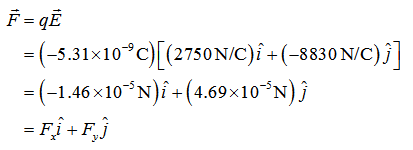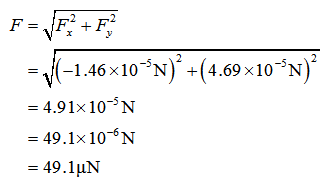# What are the magnitude and the direction of the force on a -5.31 nC charge in an electric field of +2750 N/C i^-8830 N/C j^? Answer is 49.1uN 107.3 w respect to the x axis using F=qE i^ means up(?) what does j^ mean?  How is this set up?Thanks

Question
15 views

What are the magnitude and the direction of the force on a -5.31 nC charge in an electric field of +2750 N/C i^-8830 N/C j^?

Answer is 49.1uN 107.3 w respect to the x axis using F=qE

i^ means up(?) what does j^ mean?  How is this set up?

Thanks

check_circle

star
star
star
star
star
1 Rating
Force is given by,Explanation for unit vectors:

In the above expression, i and j represent unit vectors along x and y directions respectively.

Magnitude of the force is,...

### Want to see the full answer?

See Solution

#### Want to see this answer and more?

Solutions are written by subject experts who are available 24/7. Questions are typically answered within 1 hour.*

See Solution
*Response times may vary by subject and question.
Tagged in

### Physics